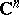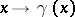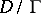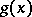# Automorphic form

A meromorphic function on a bounded domainof the complex spacethat, for some discrete group of transformationsoperating on this domain, satisfies an equation:Hereis the Jacobian of the mappingandis an integer known as the weight of the automorphic form. If the groupacts fixed-point free, then automorphic forms define differential forms on the quotient spaceand vice versa. Automorphic forms may be used in the construction of non-trivial automorphic functions (cf. Automorphic function). It has been shown that ifis a function that is holomorphic and bounded on a domain, then the seriesconverges for large values of, thus representing a non-trivial automorphic function of weight. These series are called Poincaré theta-series.